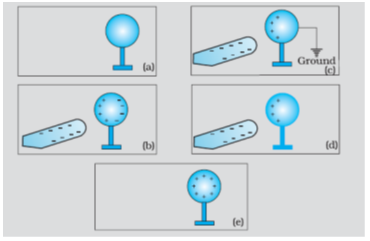# Electric Charges and Fields - Online Test

Q1. Which of the following methods can be used to charge a metal sphere positively without touching it . Select the best
Explaination / Solution:

This can be possible by induction, if a negative charge rod is placed near the sphere (at a distance not in touch) than it attracts positive charge inside the sphere towards its side and the negative charges collected on other side and when the surface of sphere connected to earth than the negative charge goes to earth and the sphere remain positively charged.Q2. If $1{0}^{9}$ electrons move out of a body to another body every second, how much time is approximately required to get a total charge of 1 C on the other body?
Explaination / Solution:

We know that 1 Coulomb = 6.242×1018 eletrons

given 109 electrons take 1 secs

=> 109 electrons ------> 1 sec

=> 1 electrons ---------> $\frac{1}{{10}^{9}}$ secs

=> 6.242×1018 ------> $\frac{6.242×{10}^{18}}{{10}^{9}}$

= 6.242×109 secs =$\frac{6.242×{10}^{9}}{60×60×24×365}$  years

=197.93 years= 200 years approx

Q3. Electric charge
Explaination / Solution:

Every matter is made up of atoms which contains protons, electrons and neutrons. Protons are +ve charged particles and electrons are - ve charged particles. Because of electric charge these particles experience a force in electrical fields.

Q4. Electric charges are of
Explaination / Solution:

Two types

• Positivecharge—deficiency of electrons i.e. if a body loses electrons it will be positive charged.
• Negative charge—excess of electrons i.e. if a body gains electrons it will be negative charged

Q5. Conservation of charges in tribo electric charging
Explaination / Solution:

The triboelectric effect (also known as triboelectric charging) is a type of contact electrification in which certain materials become electrically charged after they come into frictional contact with a different material. Rubbing glass with fur, or a plastic comb through the hair, can build up triboelectricity.

When we charge something with static electricity, no electrons are made or destroyed. No new protons appear or disappear. Electrons are just moved from one place to another. The net, or total, electric charge stays the same. This is called the principle of conservation of charge.

If a glass rod is rubbed with silk, some electrons moves from glass rod to silk no new charge is generated or destroyed.

Q6. Two positive charges
Explaination / Solution:

Acc to coulomb's law unlike charges always attract each other while like charges always repel each other so between two positive charges repulsion is working.

Q7. Charge is quantized means
Explaination / Solution:

Total charge transferred from one body to other can be calculated by the number of electrons it is known as quantisation of charge given by q=±ne, where n= number of electrons , which should be an integer.

Q8. Ionization of a neutral atom is the
Explaination / Solution:

It is not possible to remove or add protons to atom, but electron can be added or removed by an atom easily so charge can be developed on an atom by removing or adding electrons, by adding electrons it becomes negative charged ,by removing electrons it becomes positive charged.

Q9. Electrostatic force is
Explaination / Solution:

Electrostatics means charges at rest,or relatively at rest so this force can be applicable only when charge produce electric field which is constant which is possible only when charges at rest or relatively at rest.

Q10. Conductors are materials
Explaination / Solution:

Conductors are materials that permit electrons to flow freely from particle to particle. An object made of a conducting material will permit charge to be transferred across the entire surface of the object. In conductors some electrons are unpaired so these electrons can move freely.